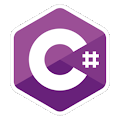Programming Course in C# ¡Free!

# Basic calculator. Several operations

Write a basic calculador in C#  to perform a basic operations. The user can only enter a number on the screen. Ask the user for a number and then perform addition, subtraction, multiplication and division of the same number.

### Input

Enter a number: 4

4 + 4 = 8
4 - 4 = 0
4 x 4 = 16
4 / 4 = 1